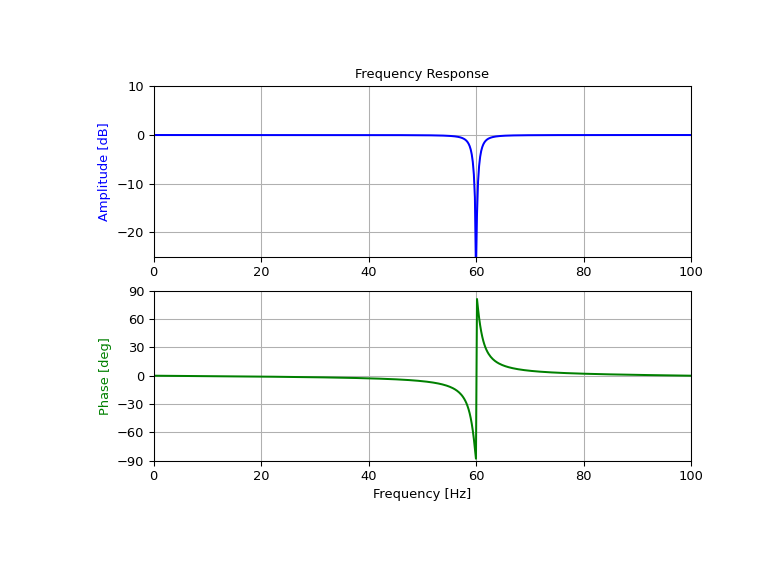scipy.signal.iirnotch¶

scipy.signal.iirnotch(w0, Q, fs=2.0)[source]

Design second-order IIR notch digital filter.

A notch filter is a band-stop filter with a narrow bandwidth (high quality factor). It rejects a narrow frequency band and leaves the rest of the spectrum little changed.

Parameters
w0float

Frequency to remove from a signal. If fs is specified, this is in the same units as fs. By default, it is a normalized scalar that must satisfy 0 < w0 < 1, with w0 = 1 corresponding to half of the sampling frequency.

Qfloat

Quality factor. Dimensionless parameter that characterizes notch filter -3 dB bandwidth bw relative to its center frequency, Q = w0/bw.

fsfloat, optional

The sampling frequency of the digital system.

New in version 1.2.0.

Returns
b, andarray, ndarray

Numerator (b) and denominator (a) polynomials of the IIR filter.

iirpeak

Notes

New in version 0.19.0.

References

1

Sophocles J. Orfanidis, “Introduction To Signal Processing”, Prentice-Hall, 1996

Examples

Design and plot filter to remove the 60 Hz component from a signal sampled at 200 Hz, using a quality factor Q = 30

>>> from scipy import signal
>>> import matplotlib.pyplot as plt
>>> fs = 200.0  # Sample frequency (Hz)
>>> f0 = 60.0  # Frequency to be removed from signal (Hz)
>>> Q = 30.0  # Quality factor
>>> # Design notch filter
>>> b, a = signal.iirnotch(f0, Q, fs)
>>> # Frequency response
>>> freq, h = signal.freqz(b, a, fs=fs)
>>> # Plot
>>> fig, ax = plt.subplots(2, 1, figsize=(8, 6))
>>> ax.plot(freq, 20*np.log10(abs(h)), color='blue')
>>> ax.set_title("Frequency Response")
>>> ax.set_ylabel("Amplitude (dB)", color='blue')
>>> ax.set_xlim([0, 100])
>>> ax.set_ylim([-25, 10])
>>> ax.grid()
>>> ax.plot(freq, np.unwrap(np.angle(h))*180/np.pi, color='green')
>>> ax.set_ylabel("Angle (degrees)", color='green')
>>> ax.set_xlabel("Frequency (Hz)")
>>> ax.set_xlim([0, 100])
>>> ax.set_yticks([-90, -60, -30, 0, 30, 60, 90])
>>> ax.set_ylim([-90, 90])
>>> ax.grid()
>>> plt.show()